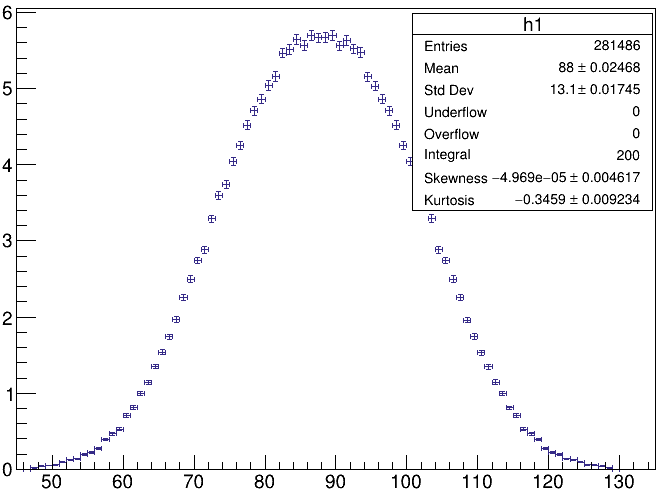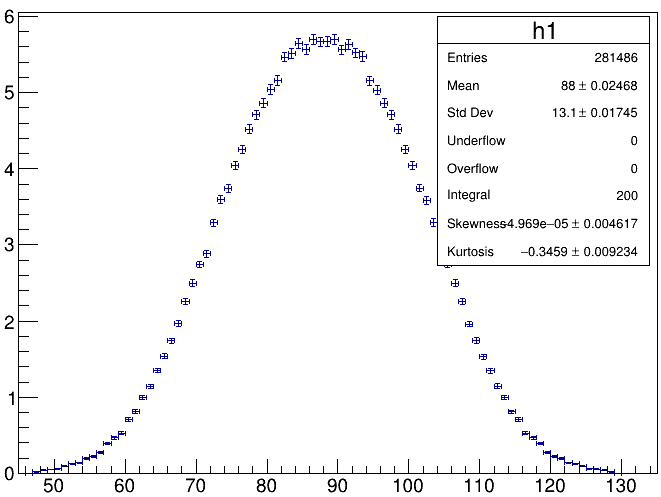# Error calculation in ROOT

Dear Sir,
I want to know how the errors are calculated by ROOT histo.

I have checked the classes responsible for the error, where I find that the the size of the error bars is equal to the square root of the number of entries in that histogram bin. That is, if a histogram bin contains “N” no. of entries the the error associated is sqrt(N). But in that case more statistics impliy more errors, which is unphysical.

But as per my understanding 1/sqrt(N) should be the error. Please let me know whether I am wrong ???

My second quary is about the option h->Sumw2();

" which means “sum the squares of the weights”" - could you please elaborate this line which I got from the manual. And in which situation should I use this option.

Regards-
Kalyan

1 Like

Hi,

If the histogram represent counts, thus when each entry that is filled has a weight=1, the statistical error in each bin is computed as SQRT(N), where N is the number of entries in each bin, which by definition is in this case equal to the bin content.

If you are filling the histogram with a weight different than one, then the bin content is equal to the sum of the weight in each bin (different than the number of entries in each bin).
The statistical error in this case con be approximately computed from SQRT( sum of weight^2) in each bin.
However, in order to have the histogram doing this, you ned to set the option TH1::Sumw2().
By doing this the histogram will store the bin sum of weight square, that can be used to compute the errors.

So you should use this option when your histogram is filled with weights different than one.

Best Regards

Lorenzo

2 Likes

Hi,

I have a similar doubt regarding the calculation of the errors of a histogram. To elaborate more, I have a histogram :

which is plotted from a ttree.

I scale the histogram to 200% using “200/h1->GetEntries()” and plots it and obtain :So my doubt is why are the error bars at the extremities so small and the error bars at the peak of the histogram large? I am interested in having the statistical uncertainty at each point for the histogram which will be larger at the extremities of the histogram (due to fewer entries), but this is not the case here. So how can I have realistic statistical uncertainty at each point of the histogram (which is quite essential to consider when I do the fitting for the histogram). Since I new to root can someone help me how to do this ?

Try first: `htemp->Draw("E1");`The histogram is plotted using the error E1 option.

htemp`->Draw("E1");`

So, the content and the errors have been properly scaled. No problem whatsoever.

But why are the errors at the peak of the histogram larger than the ones at the extremities? This is contrary to the expectation of large error bars at the extremities (due to low counts). I want to have the statistical uncertainty at each point on the histogram (which should be smaller at the peak and larger at the end of the histogram).

Usually, including your case, “`error = sqrt(content)`” (i.e., bigger “`content`”, bigger “`error`”).

I see. So is this the only way of calculating errors in the histogram …

You can use, e.g., TH1::SetBinError to set whatever you like.